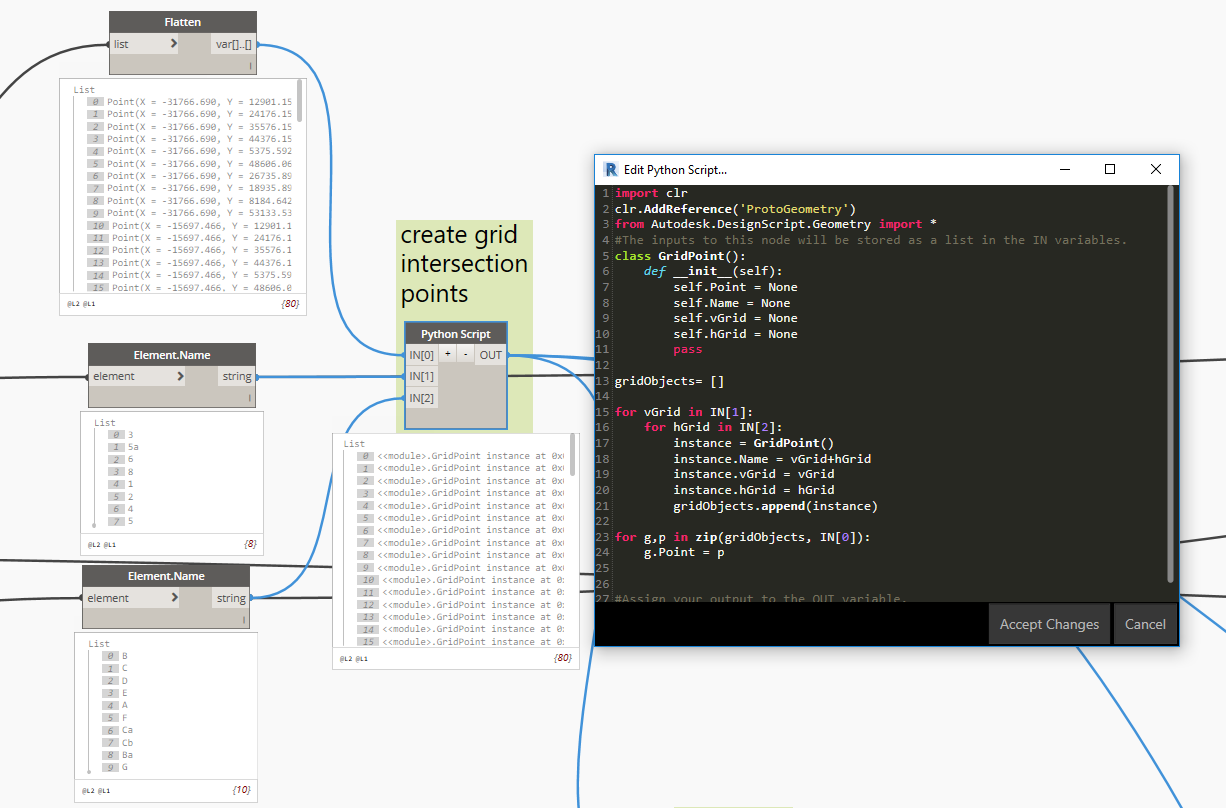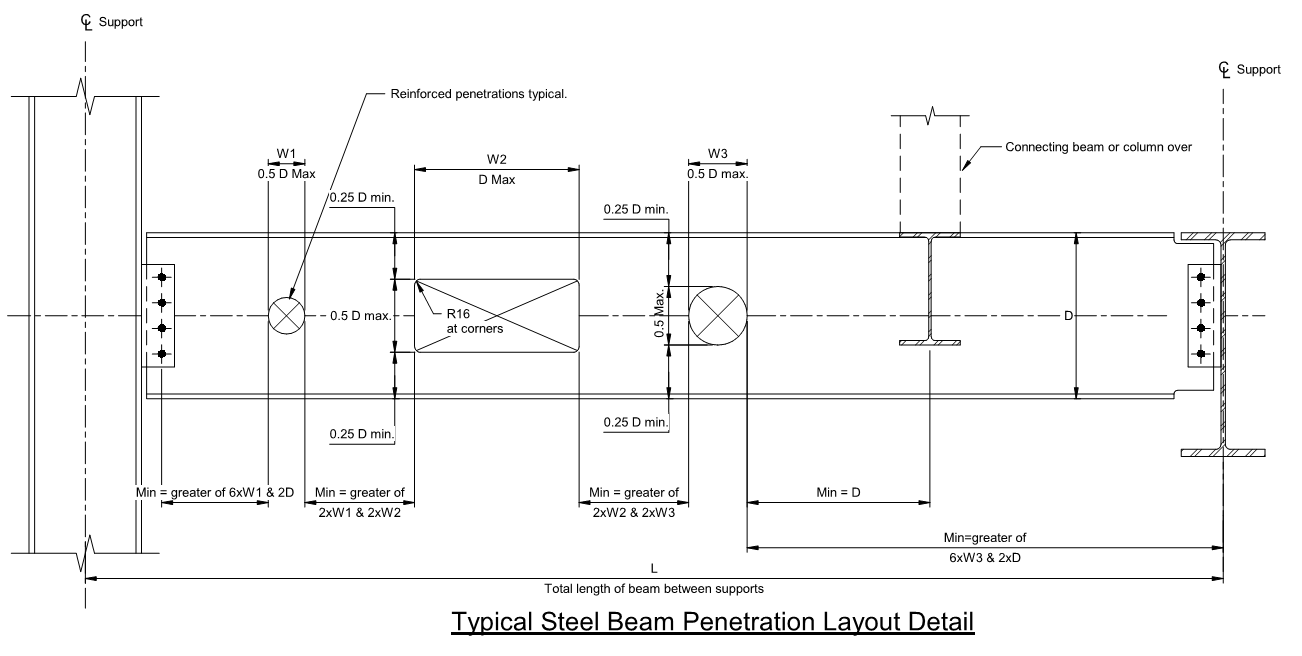# Using Python Functions and Classes

## Example 1: Find beam closest Grids

1. Find all the grid intersection points and name them accordingly (1A, 1B, 2A, 2B…). Store them into a Python class or a Dictionary.
2. Get the beam Start and End points and return the minimum distance to the grid object.

Method 1:

``````class GridPoint:
pass
#create an instance and add values
instance = GridPoint()
instance.pt = Point.ByCoordinates(0,0)
instance.Name = "1A"
``````

Method 2:

``````class GridPoint:
def __init__(self):
self.Point = None
self.Name = None
self.vGrid = None
self.hGrid = None
#create an instance and add values
instance = GridPoint()
instance.point = Point.ByCoordinates(0,0)
instance.name = "1A"
``````

The 2 methods above do not prevent the user to misspell a variable name. To force value into pre-established variables we can use a constructor:

``````class GridPoint:
def __init__(self, point, name, vGrid, hGrid):
self.pt = point
self.n = name
self.vG = vGrid
self.hG = hGrid
#create an instance using the provided constructor
instance = GridPoint(Point.ByCoordinates(0,0),"1A","1","A")
``````

Instances can be created inside a loop and appended to a list. Values of an instance can be changed after its creation:

``````list= []
for vGrid in IN:
for hGrid in IN:
instance = GridPoint(None, str(vGrid)+str(hGrid),vGrid, hGrid)
list.append(instance)

for l,p in zip(list, IN):
l.Point = p
``````Now we can check the distance between our beam end points and the grid points. If we store the distances and the grid point names together, we can retrieve the closest grid points using the minimum function.

``````import clr
from Autodesk.DesignScript.Geometry import Point

beamEnds = [IN.PointAtParameter(0),IN.PointAtParameter(1)]
gridObj = IN

distances = []

for b in beamEnds:
subList = []
distances.append(subList)
for g in gridObj:
subList.append([b.DistanceTo(g.pt),g.name])

OUT = [min(d) for d in distances]
``````## Example 2: Check that the openings in a beam are consistent with a typical detailTo check the openings we will need a list including the opening width and depth, its centroid, the beam height, the beam start and end point.

This can be achieved through a nested for loop:

``````
``````
Written on May 21, 2018